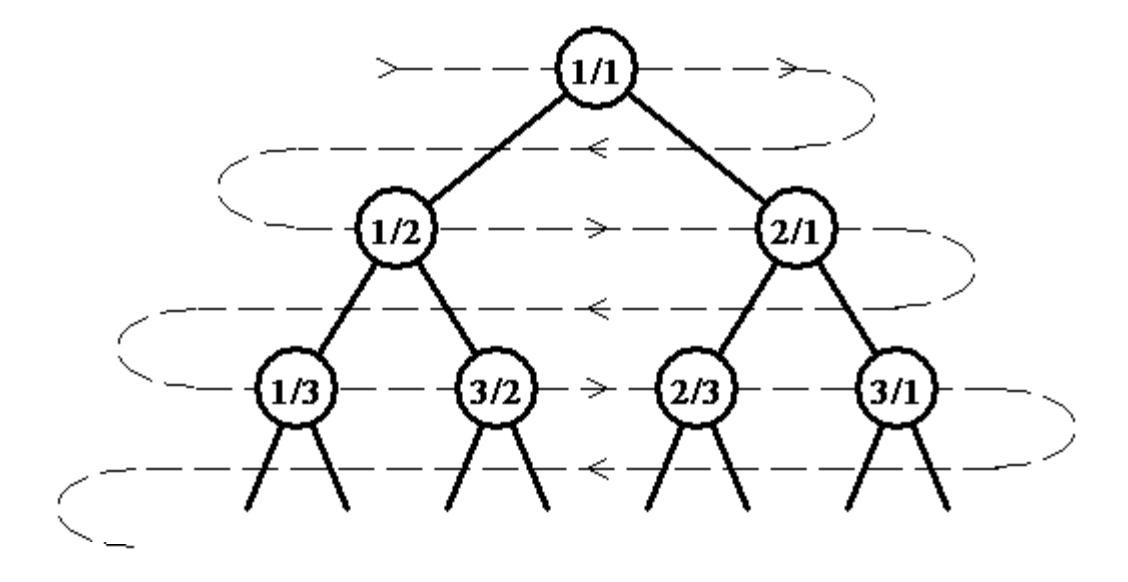시간 제한 메모리 제한 제출 정답 맞은 사람 정답 비율
1 초 256 MB 45 41 33 89.189%

## 문제

A sequence of positive rational numbers is defined as follows:

An infinite full binary tree labeled by positive rational numbers is defined by:

• The label of the root is 1/1.
• The left child of label p/q is p/(p+q).
• The right child of label p/q is (p+q)/q.

The top of the tree is shown in the following figure:The sequence is defined by doing a level order (breadth first) traversal of the tree (indicated by the light dashed line). So that:

F(1) = 1/1, F(2) = 1/2, F(3) = 2/1, F(4) = 1/3, F(5) = 3/2, F(6) = 2/3, …

Write a program which finds the value of n for which F(n) is p/q for inputs p and q.

## 입력

The first line of input contains a single integer P, (1 ≤ P ≤ 1000), which is the number of data sets that follow. Each data set should be processed identically and independently.

Each data set consists of a single line of input. It contains the data set number, K, a single space, the numerator, p, a forward slash (/) and the denominator, q, of the desired fraction.

## 출력

For each data set there is a single line of output. It contains the data set number, K, followed by a single space which is then followed by the value of n for which F(n) is p/q. Inputs will be chosen so n will fit in a 32-bit integer.

## 예제 입력 1

4
1 1/1
2 1/3
3 5/2
4 2178309/1346269


## 예제 출력 1

1 1
2 4
3 11
4 1431655765